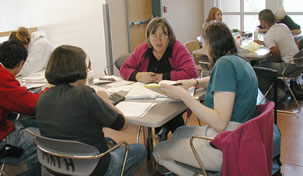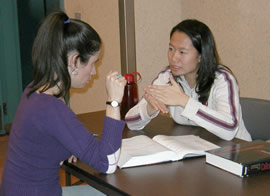# Tutoring Resources

The Mathematics and Statistics Tutoring Center offers help desks for several of our courses. A link to the hours at which a TA or instructor is available for a given course is given below.

Math 1220, 1230, 1240, 1250: Algebra Table (DSH, 2nd floor by the elevators)  [old Math 121,123,150,153]

Math 1512, 1522, 2530, 1430, 1440: Calculus Table (SMLC B-71)  [old Math 162,163, 264,180,181]

Math 1118, 2118, 2115: Math Education Table (SMLC 230) [old Math 111/1110, 215, 216]

Math 311: 300+ Table (SMLC B-71)

Math 314: 300+ Table (SMLC B-71)

Math 316: 300+ Table (SMLC B-71)

Stat 345: 300+ Table (SMLC B-71)

Math 1350: Statistics Table [old Stat 145], students should check their LaunchPad online course materials for information